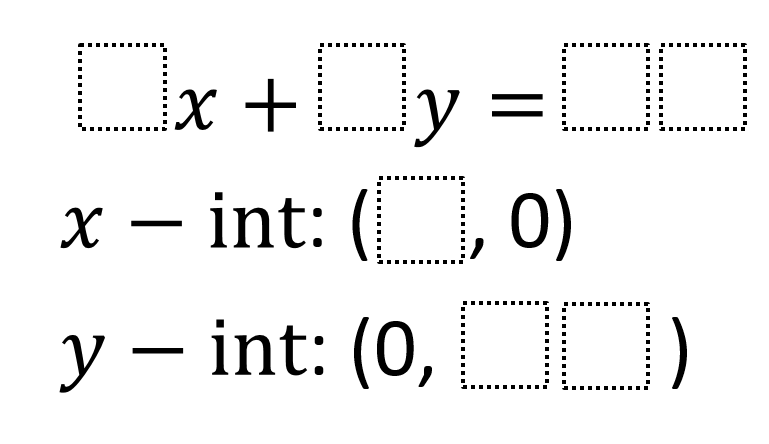# Intercept Form Equations

Directions: Using digits 1-9, write an equation of a line in standard form with given x- and y-intercepts. Each number can only be used at most once.### Hint

What numbers can we rule out as coefficients for x and y in the equation?